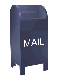### The RC5 Algorithm modified to mirror some details of hardware implementation

```
/*
Copyright (C) 1995 RSA Data Security, Inc.

This code is available from RSA under the conditions that it is not
used in commercial products without RSA's written permission.  This
code is also made available with the provision that it not be
exported outside the United States, since the pinheads in the
government consider this a munition.  This web page is only for
those within the United States.  So if you are reading this web

Oh, and if you're not within the United States please be sure to
avoid reading Bruce Schneier's book Applied Cryptography:
Protocols, Algorithms, and Source Code in C, 2nd Edition, since
this book contains cryptographic algorithms you should not see.
Thank you for making the job of ECHELON easier.

As they say at the NRA: "when cryptography is outlawed, only
outlaws will have cryptography".

This code is an ANSI C rewrite of the RC5REF.C reference
implementation of the RC5-32/12/16 cryptographic algorithm.
Rewrite by Ian Kaplan, June 1995.  This code has been written as a
model for a Hardware Design Language implementation of this
algorithm.  To make hardware implementation more practical, the
algorithm has been reduced to shifts and adds.  The number of
"rounds" has been increased from 12 to 15, to get rid of a mod "%"
operation in the setup function.

Ian Kaplan
iank@bearcave.com

*/

#include <stdio.h>
#include <time.h>

#define KEYSIZE 16	 /* size of key, in bytes */
typedef unsigned int WORD; /* Should be 32-bit = 4 bytes        */

typedef enum { ShiftLeft, ShiftRight } ShiftDir;

typedef enum { KeyWords = KEYSIZE / 4,
NumRounds = 15,  /* Number of cryptographic rounds  */
TableSize = 32   /* size of table = 2 * (NumRounds + 1) */
} bogus;

WORD Table[ TableSize ];
WORD L[KeyWords];

WORD ROT(const WORD x, const WORD y, const ShiftDir dir)
{

const unsigned int ShiftAmt = (y & (unsigned int)0x1f);
const unsigned int ShiftBack = 0x20 - ShiftAmt;
unsigned int result;

if (dir == ShiftLeft)
result = (x << ShiftAmt) | (x >> ShiftBack);
else
result = (x >> ShiftAmt) | (x << ShiftBack);
return result;
} /* ROT */

void SetKey( unsigned char KeyChar )
{
static unsigned int KeyCntr;
static unsigned int Shift;

int ix = KeyCntr >> 2;

/* this is simply a machine independent way of setting L[i] to
KeyChar[i], without being affect by "endianess". */
L[ ix ] = (L[ ix ] & (~(0xff << Shift))) | (KeyChar << Shift);

Shift = (Shift + 8) & 0x1f;
KeyCntr = (KeyCntr + 1) & (KEYSIZE - 1);  /* This Depends on KEYSIZE being */
/* a power of two.  The & will   */
/* cause the KeyCntr to wrap     */
/* and only have values in the   */
/* range 0..KEYSIZE-1.           */
}  /* RC5_Crypto */

/* 2 WORD: input plain text, output encrypted text    */
void encrypt(WORD *PlainText, WORD *CryptoText)
{

WORD i, temp;
WORD A;
WORD B;

A = PlainText + Table;
B = PlainText + Table;

for (i = 1; i <= NumRounds; i++) {
temp = i << 1;
A = ROT(A^B, B, ShiftLeft) + Table[temp];
B = ROT(A^B, A, ShiftLeft) + Table[temp+1];
}
CryptoText = A;
CryptoText = B;
}  /* RC5_Cypto::encrypt */

/* 2 WORD input encrypted text, output plain text    */
void decrypt(WORD *CryptoText, WORD *PlainText)
{
WORD i, temp;
WORD B;
WORD A;

B = CryptoText;
A = CryptoText;

for (i=NumRounds; i > 0; i--) {
temp = i << 1;
B = ROT(B - Table[temp+1],A, ShiftRight)^A;
A = ROT(A - Table[temp],  B, ShiftRight)^B;
}
PlainText = B-Table;
PlainText = A-Table;
} 	/*  decrypt */

void setup() /* secret input key K[0...KEYSIZE-1]   */
{
/* magic constants (courtesty of RSA) */
static const WORD ROM[ TableSize ] = { 0xb7e15163, 0x5618cb1c, 0xf45044d5,
0x9287be8e, 0x30bf3847, 0xcef6b200,
0x6d2e2bb9, 0x0b65a572, 0xa99d1f2b,
0x47d498e4, 0xe60c129d, 0x84438c56,
0x227b060f, 0xc0b27fc8, 0x5ee9f981,
0xfd21733a, 0x9b58ecf3, 0x399066ac,
0xd7c7e065, 0x75ff5a1e, 0x1436d3d7,
0xb26e4d90, 0x50a5c749, 0xeedd4102,
0x8d14babb, 0x2b4c3474, 0xc983ae2d,
0x67bb27e6, 0x05f2a19f, 0xa42a1b58,
0x42619511, 0xe0990eca };
WORD i;
WORD A;
WORD B;
WORD j;
WORD k;

/* Copy "ROM" into "RAM" */
for (i=0; i < TableSize; i++)
Table[i] = ROM[i];

/* 3*t > 3*KeyWords */

A = 0;
B = 0;
i = 0;
j = 0;

for (k=0; k < 3*TableSize; k++) {
Table[i] = ROT(Table[i]+(A+B),3, ShiftLeft);
A = Table[i];
L[j] = ROT(L[j]+(A+B),(A+B), ShiftLeft);
B = L[j];
i= (i+1) & (TableSize-1);  /* using '&' for % only works for powers of 2  */
j= (j+1) & (KeyWords-1);
}
} 	/* setup */

/*
Testbench for crytography algorithm
*/
void main()
{
WORD i, j;
WORD PlainText1, PlainText2;
WORD CryptoText = {0,0};
time_t t0, t1;
char *keystr = "TheQuickBrownFox";

if (sizeof(WORD)!=4)
printf("RC5 error: WORD has %d bytes.\n",sizeof(WORD));

printf("RC5-32/12/16 examples:\n");
for (i = 1; i<6; i++)
{
PlainText1 = CryptoText;
PlainText1 = CryptoText;
for (j=0; j < KEYSIZE; j++)
SetKey((unsigned char)keystr[j]);
/* Setup, encrypt, and decrypt */
setup();
encrypt(PlainText1,CryptoText);
decrypt(CryptoText,PlainText2);

/* Print out results, checking for decryption failure */
printf("\n   plaintext %.8lX %.8lX  --->  ciphertext %.8lX %.8lX  \n",
PlainText1, PlainText1, CryptoText, CryptoText);
if (PlainText1!=PlainText2 || PlainText1!=PlainText2)
printf("Decryption Error!");
}
time (&t0);
for (i=1;i<100000;i++)
encrypt(CryptoText,CryptoText);
time (&t1);
printf ("\n   Time_t for 100000 blocks:  %ld \n", t1-t0);
}

```

back to C as a hardware design language